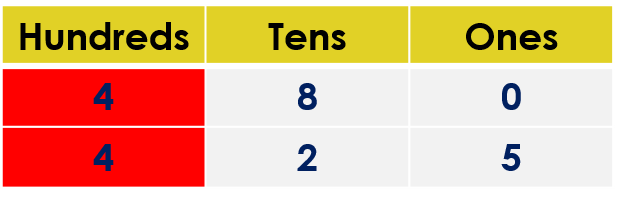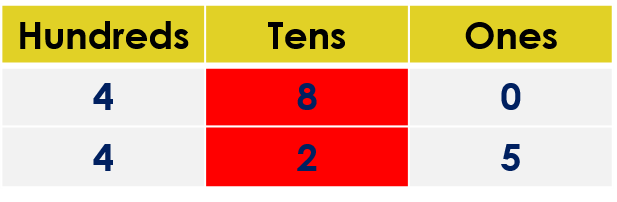1
visibility
Fill in the blank space with the correct sign. Use either <, > or =.
480  \$latex \framebox[0.5in]{}\$  425

The correct answer is ">".

It is easy!

• Compare the hundreds digits.
• If the hundreds digits are the same, then compare the tens digits.
• If tens digits are the same, then compare the ones digits.

Solution:

Observe that both numbers have three digits.

480 ____ 425

Comparing the hundreds digits:We observe that both digits are the same.

Comparing the tens digits:We observe that both digits are NOT the same.

• The tens digit in the number 480 is 8.
• The tens digit in the number 425 is 2.

But, 8 is greater than 2.

So, 480 is greater than 425.

That is, 480 > 425.